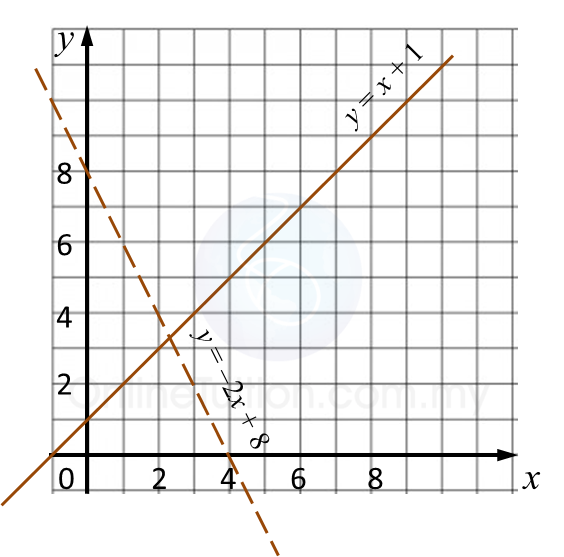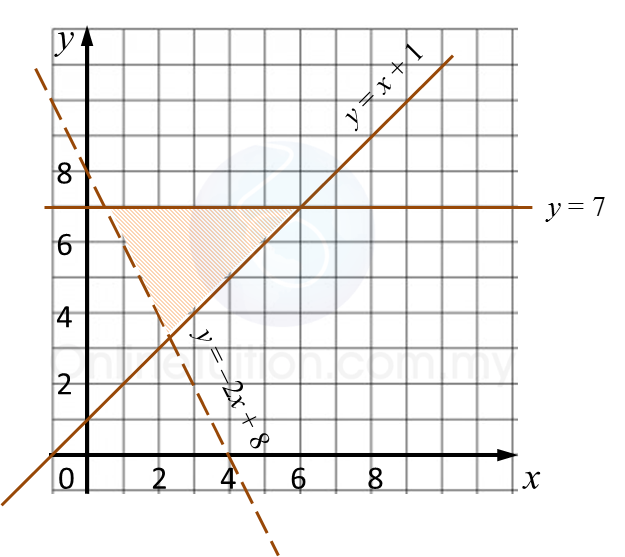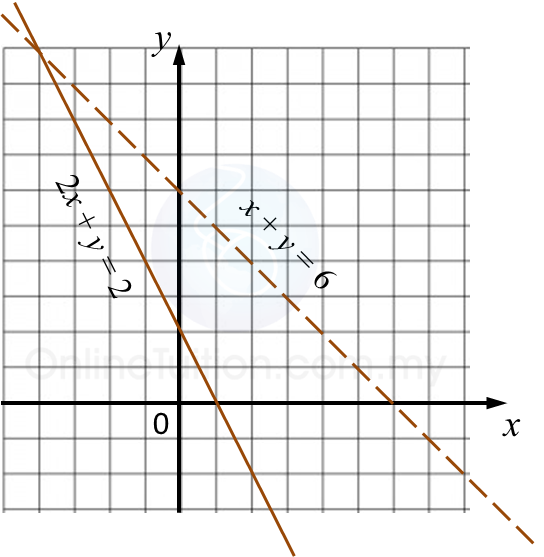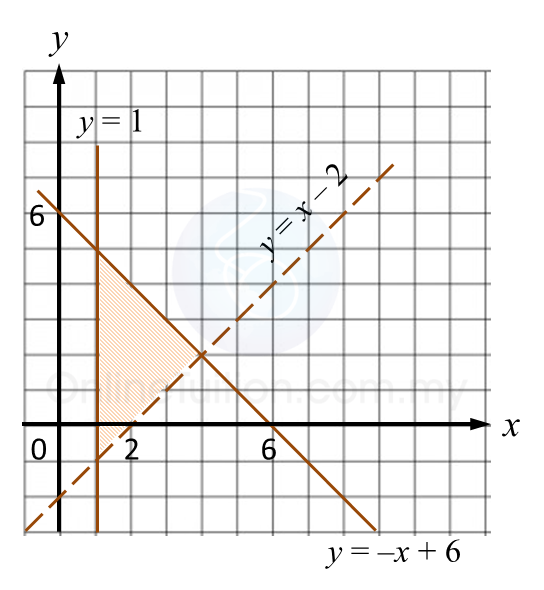# 6.4.1 Linear Inequalities in Two Variables, SPM Paper 2 (Long Questions)

Question 1:
On the graph in the answer space, shade the region which satisfies all three inequalities y > –2x + 8, yx + 1 and y ≤ 7.Solution:Question 2:
On the graph in the answer space, shade the region which satisfies all three inequalities 2x + y ≥ 2, x + y < 6 and y ≥ 1.Solution
:Question 3:
On the graph in the answer space, shade the region which satisfies all three inequalities yx – 2, y > –3x + 6 and y ≤ 4.Solution:Question 4:
On the graph in the answer space, shade the region which satisfies all three inequalities y > x – 2, y ≤ –x + 6 and x ≥ 1.1.